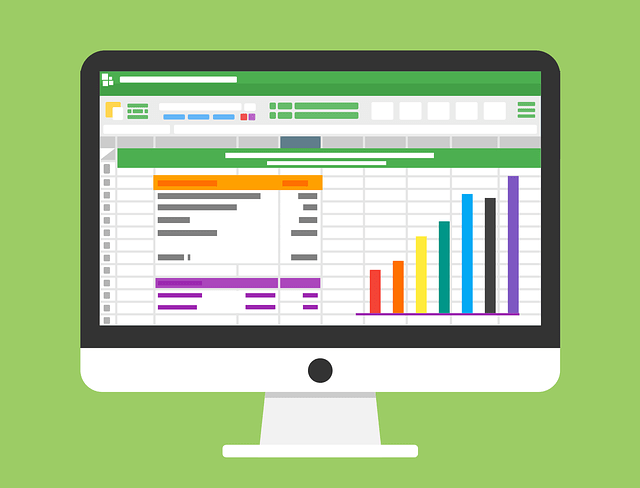### Average Last 3 Customer Sales: Power Query, DAX Measure or Worksheet Formula

Download Excel Finished Files: http://bit.ly/2K9SNfn
Entire page with all Excel Files for All Videos: http://bit.ly/1kSFWvs

In this video learn how to Average a customers last three sales (last three dates) using Excel Worksheet Formulas, Power Query M Code and a Power Pivot & Power BI DAX Measure.
Topics:
1. (00:07) Introduction
2. (00:50) Sort Method
3. (02:09) Excel Worksheet Formulas, including AGGREGATE and AVERAGEIFS functions.
4. (05:27) LARGE Function and New Excel Office 365 Calculation Engine
5. (08:15) Power Query M Code, including Table.Sort, List.Average and List.FirstN M Code functions
6. (12:46) Power Query Parameter Query with condition coming from Excel Worksheet
7. (14:05) Power Pivot & Power BI DAX Measure, including TOPN, CALCULATE and AVERAGE DAX Functions
8. (17:08) DAX Parameter from Excel Worksheet using VALUES DAX Function.
9. (18:43) Add new records to table and test formulas
10. (19:00) Fix Power Query Table.Sort bug using Dummy Insert Column
11. (20:08) Fix Power Query Table.Sort bug using Table.Sort function inside Table.Group Function
12. (22:12) Conclusion

View on YouTube### Comprehensive Introduction to Excel Power Pivot, DAX Formulas and DAX Functions

Download Excel START File: https://ift.tt/2FrxeX5
Second Excel Start File: https://ift.tt/2Dtf4Sf
Download Zipped Folder with Text Files: https://ift.tt/2Frxfu7
Download Excel FINISHED File: https://ift.tt/2qSnYkx
Download pdf Notes about Power Query: https://ift.tt/2FrxwgD
Assigned Homework:
Download Excel File with Instructions for Homework: https://ift.tt/2qRom2T
Examples of Finished Homework: https://ift.tt/2Frxgyb

### This video teaches everything you need to know about Power Pivot, Data Modeling and building DAX Formulas, including all the gotchas that most Introductory videos do not teach you!!!

Comprehensive Microsoft Power Tools for Data Analysis Class, BI 348, taught by Mike Girvin, Excel MVP and Highline College Professor.

Topics:
(00:15) Introduction & Overview of Topics in Two Hour Video
1. (04:36) Standard PivotTable or Data Model PivotTable?
2. (05:51) Excel Power Pivot & Power BI Desktop?
3. (12:31) Power Query to Extract, Transform and Load Data to Data Model – Get data from Text Files, Relational Database and Excel File.
4. (25:47) Build Relationships
5. (27:43) Introduction to DAX Formulas: Measures & Calculated Columns
6. (29:15) DAX Calculated Column using the DAX Functions, RELATED and ROUND
7. (31:20) Row Context: How DAX Calculated Columns are Calculated: Row Context
8. (33:49) We do not want to use Calculated Column results in PivotTable using Implicit Measures
9. (34:05) DAX Measure to add results from Calculated Column, using DAX SUM Function.
10. (35:29) Number Formatting for DAX Measures
11. (36:35) Data Model PivotTable
12. (39:31) Explicit DAX Formulas rather than Implicit DAX Formulas
13. (41:50) Show Implicit Measures
14. (45:00) Filter Context (First Look) How DAX Measures are Calculated
15. (50:14) Drag Columns from Fact Table or Dimension Table?
16. (53:30) Hiding Columns and Tables from Client Tool
17. (55:52) Use Power Query to Refine Data Model
18. (57:54) SUMX Function (Iterator Function). DAX Measure for Revenue using the SUMX Function to simulate Calculated Columns in DAX Measures
19. (01:01:00) Compare and Contrast Calculated Columns & Measures
20. (01:04:26) Why We Need a Date Table. Why we do NOT use the Automatic Grouping Feature for a Data Model PivotTable
21. (01:06:46) Build an Automatic Date Table in Excel Power Pivot. And then build Relationship.
22. (01:11:00) Introduction to Time Intelligence DAX Functions. See TOTALYTD DAX Function
23. (01:13:47) Introduction to CALCULATE Function: Function that can “see” Data Model and can change the Filter Context. (01:18:00) Also see the ALL and DIVIDE DAX Functions. Create formula for “% of Grand Total”. Also learn about (01:21:30) Context Transition and the Hidden CALCULATE on all Measures.
24. (01:27:18) DAX Formula Benefits.
25. (01:28:00) Example of DAX Formula that is easier to author than if we tried to do it with a Standard Pivot Table or Array Formulas
26. (01:31:50) AVERAGEX Function (Iterator Function) to calculate Average Daily Revenue.
27. (01:34:00) Filter Context (Second Look) AVERAGEX Iterator Function
28. (01:36:16) Build Dashboard. Create multiple DAX Formulas. Create Multiple Data Model PivotTables and a Data Model Chart.
29. (01:38:38) Create Measures for Gross Profit and Gross Profit %
30. (01:41:27) Continue making more Data Model PivotTables.
31. (01:41:50) Make Data Model Pivot Chart.
32. (01:45:10) Conditional Formatting for Data Model PivotTable.
33. (01:46:22) DAX Text Formula for title of Dashboard
34. (01:47:50) CUBE Function to Convert Data Model PivotTable to Excel Spreadsheet Formulas.
35. (01:50:05) Adding New Data and Refreshing.
36. (01:50:40) Update Excel Power Pivot Automatic Date (Calendar) Table. Clue is the blank in the Dimension Table Filter.
37. (01:52:20) How to Double Check that a DAX Formula is yielding the correct answer?
38. (01:53:22) DAX Table Functions. See CALCULATETABLE DAX Function.
39. (01:55:07) DAX Studio to visualize DAX Table Functions, and to efficiently create DAX Formulas
40. (02:00:12) Existing Connections to import data from Data Model into an Excel Sheet
(02:03:15) Summary

View on YouTube